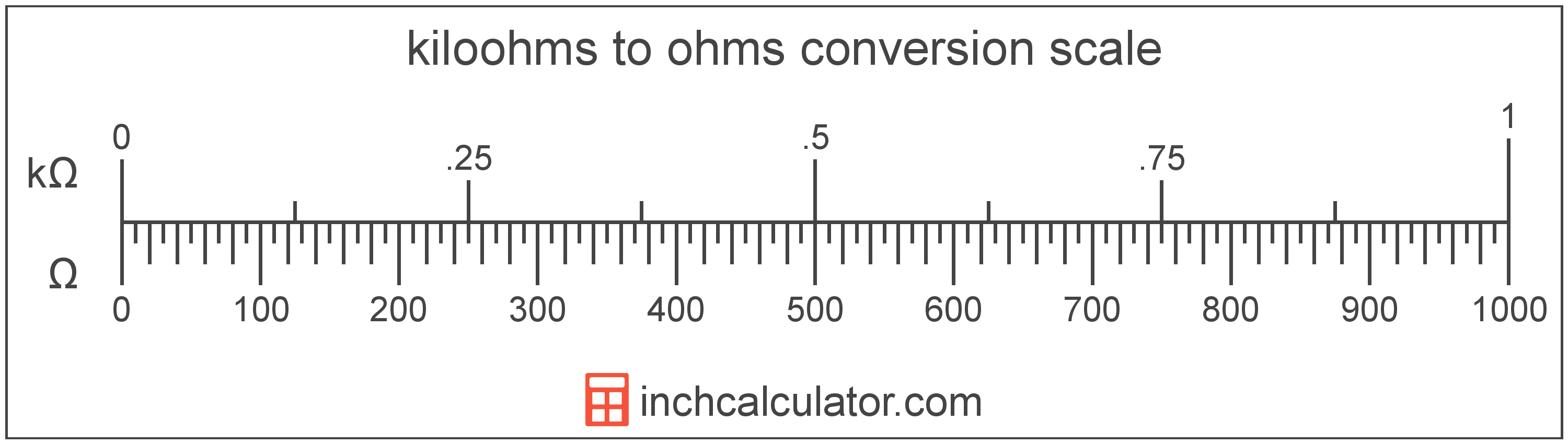# Ohms to Kiloohms Converter

Enter the electrical resistance in ohms below to get the value converted to kiloohms.

Results in Kiloohms:1 Ω = 0.001 kΩ

Do you want to convert kiloohms to ohms?

## How to Convert Ohms to Kiloohms

To convert a measurement in ohms to a measurement in kiloohms, divide the electrical resistance by the following conversion ratio: 1,000 ohms/kiloohm.

Since one kiloohm is equal to 1,000 ohms, you can use this simple formula to convert:

kiloohms = ohms ÷ 1,000

The electrical resistance in kiloohms is equal to the electrical resistance in ohms divided by 1,000.

For example, here's how to convert 5,000 ohms to kiloohms using the formula above.
kiloohms = (5,000 Ω ÷ 1,000) = 5 kΩ## What is an Ohm?

An ohm is the resistance between two points of an electrical conductor transmitting a current of one ampere when the electrical potential difference is one volt between the points.

The ohm is the SI derived unit for electrical resistance in the metric system. Ohms can be abbreviated as ; for example, 1 ohm can be written as 1 Ω.

### Ohm's Law

Ohm's Law states the current flowing between two points on a conductor is proportional to the voltage and inversely proportional to the resistance. More specifically, the current is equal to the voltage divided by the resistance.

Using Ohm's Law, it's possible to express the resistance in ohms as an expression using current and voltage.

R = VV / IA

Here, the resistance R in ohms is equal to the potential difference V in volts divided by the current I in amperes.

## What is a Kiloohm?

One kiloohm is equal to 1,000 ohms. A kiloohm is the resistance across a conductor with an electrical potential difference of 1,000 volts with one ampere of current flowing through it.

The kiloohm is a multiple of the ohm, which is the SI derived unit for electrical resistance. In the metric system, "kilo" is the prefix for thousands, or 103. Kiloohms can be abbreviated as kΩ; for example, 1 kiloohm can be written as 1 kΩ.

## Ohm to Kiloohm Conversion Table

Table showing various ohm measurements converted to kiloohms.
Ohms Kiloohms
1 Ω 0.001 kΩ
2 Ω 0.002 kΩ
3 Ω 0.003 kΩ
4 Ω 0.004 kΩ
5 Ω 0.005 kΩ
6 Ω 0.006 kΩ
7 Ω 0.007 kΩ
8 Ω 0.008 kΩ
9 Ω 0.009 kΩ
10 Ω 0.01 kΩ
20 Ω 0.02 kΩ
30 Ω 0.03 kΩ
40 Ω 0.04 kΩ
50 Ω 0.05 kΩ
60 Ω 0.06 kΩ
70 Ω 0.07 kΩ
80 Ω 0.08 kΩ
90 Ω 0.09 kΩ
100 Ω 0.1 kΩ
200 Ω 0.2 kΩ
300 Ω 0.3 kΩ
400 Ω 0.4 kΩ
500 Ω 0.5 kΩ
600 Ω 0.6 kΩ
700 Ω 0.7 kΩ
800 Ω 0.8 kΩ
900 Ω 0.9 kΩ
1,000 Ω 1 kΩ

## References

1. International Bureau of Weights and Measures, The International System of Units, 9th Edition, 2019, https://www.bipm.org/documents/20126/41483022/SI-Brochure-9-EN.pdf